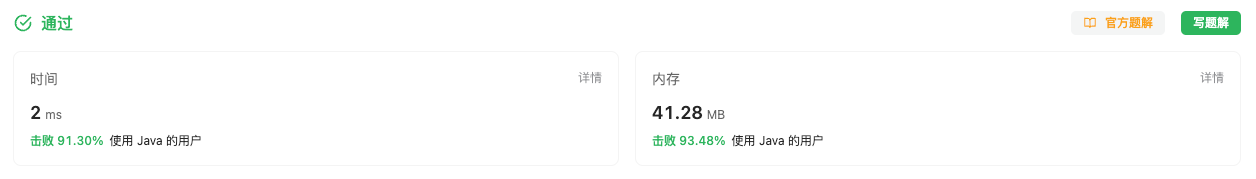#### LCP 50. 宝石补给

###### 来源: 每日一题 2023.09.15

``````输入：gem = [3,1,2], operations = [[0,2],[2,1],[2,0]]

``````

``````输入：gem = [100,0,50,100], operations = [[0,2],[0,1],[3,0],[3,0]]

``````

``````输入：gem = [0,0,0,0], operations = [[1,2],[3,1],[1,2]]

``````

• `2 <= gem.length <= 10^3`

• `0 <= gem[i] <= 10^3`

• `0 <= operations.length <= 10^4`

• `operations[i].length == 2`

• `0 <= operations[i], operations[i] < gem.length`

``````class Solution {
public int giveGem(int[] gem, int[][] operations) {

}
}
``````

#### 分析与题解

• 模拟法

对于这个题目来说, 解题思路很简单, 我们只需要进行模拟宝石的给与过程, 然后遍历一遍宝石的各个勇者持有的数量即可.

首先就是宝石的给与过程, 整体比较简单, 代码如下所示.

``````for(int[] operation : operations) {
gem[operation] = gem[operation] / 2 + gem[operation];
gem[operation] = gem[operation] - gem[operation]/2;
}
``````

然后就是定义两个常量, 分别是 `max``min`, 用于存储勇者中的持有对多的宝石数和最少的宝石数.

``````int max = Integer.MIN_VALUE;
int min = Integer.MAX_VALUE;
for(int number : gem) {
max = Math.max(max, number);
min = Math.min(min, number);
}
``````

两数做差即可得出最终结果.

``````return max - min;
``````

整体逻辑代码如下所示.

``````class Solution {
public int giveGem(int[] gem, int[][] operations) {
// 模拟法
for(int[] operation : operations) {
gem[operation] = gem[operation] / 2 + gem[operation];
gem[operation] = gem[operation] - gem[operation]/2;
}
int max = Integer.MIN_VALUE;
int min = Integer.MAX_VALUE;
for(int number : gem) {
max = Math.max(max, number);
min = Math.min(min, number);
}
return max - min;
}
}
``````

复杂度分析:

• 时间复杂度: O(m + n), m 是 gem 的长度, n 是 operations 的长度, 需要对两者都进行一次遍历.
• 空间复杂度: O(1), 常量级别的空间复杂度.

结果如下所示.IT界无底坑洞栋主 欢迎加Q骚扰:676758285